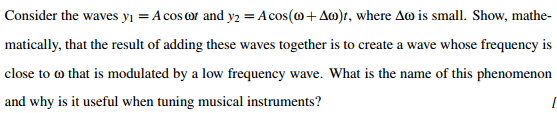# Sound (harmonic) waves questions

Hello,
I have two question regrading sound waves.
The first one:
The pressure P(x;t) at a point x at time t in a medium through which a harmonic wave is
travelling can be described by:
P(x,t) = Asin(wt -kx)
If the equation describes a pressure wave travelling in air, with amplitude 2 Pa and frequency 100 Hz,
find the instantaneous pressure at a distance 10 cm from the source at time 5s.
My solution(am I doing right?):
w=2pi*f

k=2pi/λ; v=f*λ; λ=343m/s /100 Hz = 3.43m and k=2pi/3.43 =1.83 rad/m;

Finally I have this:
P(x=0.1 ,t=5) = 2sin(200pi*5 -1.18*0.1) = -0.34.

The second question is hereHope you can help me to figure out the solutions.
Thanks

NascentOxygen
Staff Emeritus
The first one:
The pressure P(x;t) at a point x at time t in a medium through which a harmonic wave is
travelling can be described by:
P(x,t) = Asin(wt -kx)
If the equation describes a pressure wave travelling in air, with amplitude 2 Pa and frequency 100 Hz,
find the instantaneous pressure at a distance 10 cm from the source at time 5s.
My solution(am I doing right?):
w=2pi*f

k=2pi/λ; v=f*λ; λ=343m/s /100 Hz = 3.43m and k=2pi/3.43 =1.83 rad/m;
I can see how you approached this, but the assumption of v = 343 m/sec seems unreasonable in light of knowing nothing more than it is sound in "a medium". I think the best you can do is state " I can't see how this can be solved without knowing v or λ so to allow computations I am going to imagine the medium is air at 25C " or something like that.

For your second problem, you'll need to use trig identities, cos (A) + cos (B)

In the end of question's paper it says 343m/sec, so I decided to use it. I'm just confused with the negative result.

NascentOxygen
Staff Emeritus
A sinusoid "with amplitude of 2 Pa" is a convention or standard expression implying pressure varies cyclically from 2 Pa below ambient (rarefaction) to 2 Pa above ambient (compression). If it were intended to be otherwise, the question would be worded to make that clear.

So the negative result translates into;
Atmospheric(Pa) + (-0.34 Pa) = Absolute (Pa) ?

NascentOxygen
Staff Emeritus
•